# 扩展欧几里德算法总结

### 引入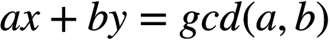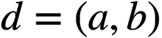，则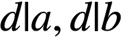。由于整除的性质，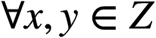，则有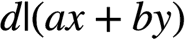。设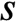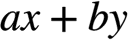的最小正值，则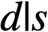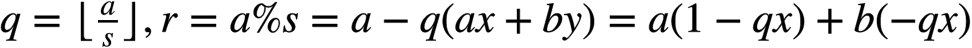所以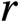也是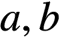的一个线性组合。由于的线性组合的最小值，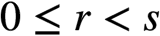，可得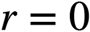。则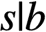，同理，则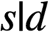，因此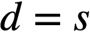，证毕。

### 流程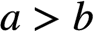1.当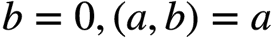，显然可得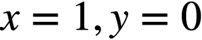2.当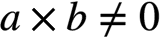时：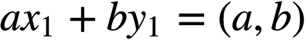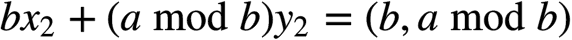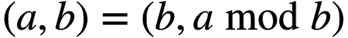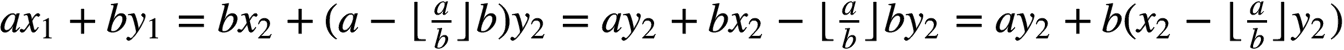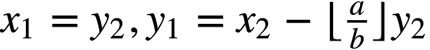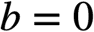时，即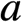为最大公因数。这时只需要保证的系数即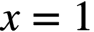即可，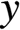的取值是没有影响的，不妨设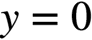### 代码

int exgcd(int a,int b,int &x,int &y)
{
if (!b)
{
x=1,y=0;
return a;
}
int ans=exgcd(b,a%b,x,y);
int t=x;
x=y;
y=t-(a/b)*y;
return ans;
}

### 应用

#### 求解丢番图方程

bool linear_equation(int a,int b,int c,int &x,int &y)
{
int d=exgcd(a,b,x,y);
if(c%d)
return false;
int k=c/d;
x*=k; y*=k;    //求得的只是其中一组解
return true;
}

#### 求解模线性方程

##### 解法一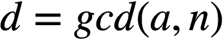，假如整数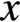，满足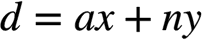(用扩展欧几里德得出)。如果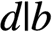，则方程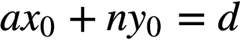，两边乘以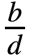，(因为，所以能够整除)，得到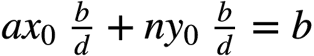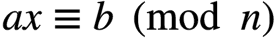的一个解为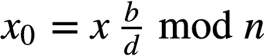，且方程的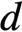个解分别为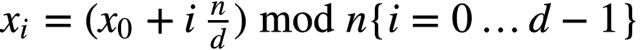##### 解法二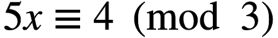;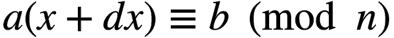;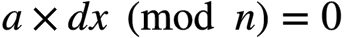;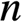的最大公约数为,那么的最小公倍数为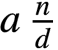.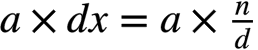;

bool modular_linear_equation(int a,int b,int n)
{
int x,y,x0,i;
int d=exgcd(a,n,x,y);
if(b%d)
return false;
x0=x*(b/d)%n;   //特解
for(i=1;i<d;i++)
printf("%d\n",(x0+i*(n/d))%n);
return true;
}

#### 求解乘法的逆元

##### 定义

//模运算法则
//
//(A + B) % C = (A % C + B % C) % C
//
//(A - B) % C = (A % C - B % C) % C
//
//(A * B) % C = (A % C) * (B % C) % C
//
//(A / B) % C = ???

##### 解法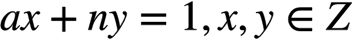。这个可用扩展欧几里德算法求出，原同余方程的唯一解就是用扩展欧几里德算法得出的int mod_inverse(int a, int m)
{
int x, y;
extgcd(a, m, x, y);
return (m + x % m) % m;
}